Function Repository Resource:

# ArcLengthIntegral

Generate an inactive integral or sum of integrals used for computing the arc length of an expression with given bounds

Contributed by: Wolfram|Alpha Math Team
 ResourceFunction["ArcLengthIntegral"][expr,{x,a,b}] returns an inactive integral for computing the arc length of expr for a≤x≤b. ResourceFunction["ArcLengthIntegral"][expr,{θ,a,b},"polar"] returns an inactive integral for computing the arc length of expr for a≤θ≤b in polar coordinates. ResourceFunction["ArcLengthIntegral"][{expr1,expr2,…},{t,a,b}] returns an inactive integral for computing the arc length of the parametric curve defined by {x1[t],x2[t],…}⩵{expr1,expr2,…} for a≤t≤b. ResourceFunction["ArcLengthIntegral"][eqtn,{{x,a,b},{y,c,d}}] returns an inactive integral for computing the arc length of the curve given by implicit equation eqtn for a≤x≤b and c≤y≤d.

## Details and Options

ResourceFunction["ArcLengthIntegral"][expr,{x,lo,hi}] accepts a math expression, an equation of the form y==f[x] or a list of two or more math expressions in terms of x.
In ResourceFunction["ArcLengthIntegral"][{expr1,expr2,},{x,lo,hi}], the expri are expected to be math expressions, not equations.

## Examples

### Basic Examples (2)

Set up the integral for computing the arc length of a sine wave:

 In:=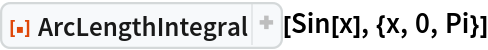Out=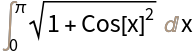Activate to compute the full result:

 In:=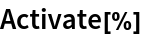Out=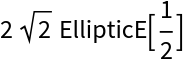Set up the integral that computes the arc length of a circle:

 In:=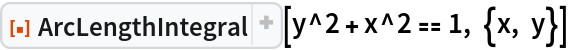Out=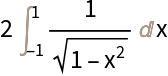### Scope (4)

Return the integral for the arc length of a curve given in polar coordinates:

 In:=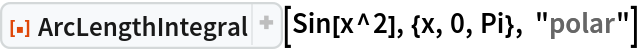Out=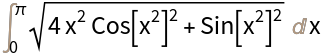Return the integral for the arc length of a parametric curve:

 In:=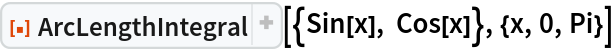Out=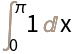Return the integral for the arc length of the unit circle that is contained within the region bounded by 0x1 and 0y1/2:

 In:=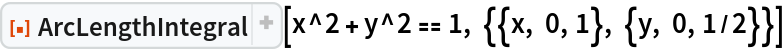Out=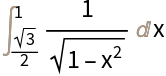You can also leave x or y unbounded:

 In:=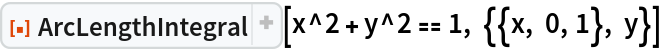Out=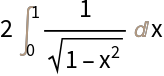Return the integral for the arc length of an asteroid bounded by -1x1:

 In:=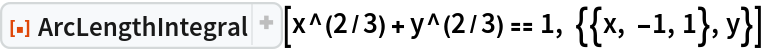Out=### Applications (3)

Define and display an implicit region representing two disconnected segments of the unit circle:

 In:=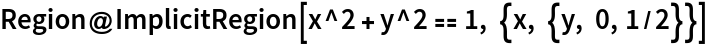Out=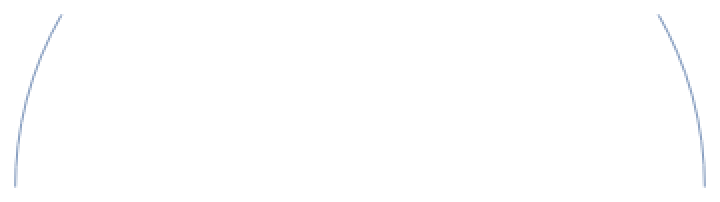Set up the integrals for disconnected segments of the region:

 In:=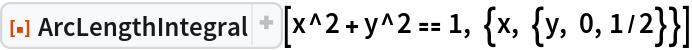Out=Then compute the result:

 In:=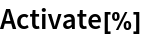Out=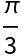### Possible Issues (1)

ArcLengthIntegral returns the integral for computing the unsigned arc length:

 In:=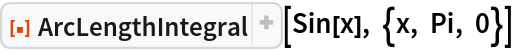Out=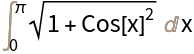## Publisher

Wolfram|Alpha Math Team

## Version History

• 2.0.0 – 23 March 2023
• 1.0.0 – 01 April 2020